↑ 收起筛选 ↑中，内角的对边分别为，则下列等式中成立的是（　　 ）

A.B.C.D.•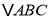中，内角A，B，C的对边分别为a，b，c，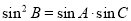.若对于任意实数，不等式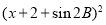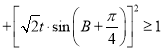恒成立，则实数t的取值范围为（　　 ）

A.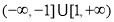B.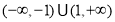C.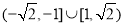D.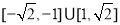高三数学单选题困难题查看答案及解析

• 下列不等式中成立的是（　　 ）

A.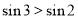B.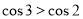C.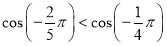D.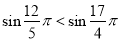高一数学单选题中等难度题查看答案及解析

• 下列不等式中成立的是（　　 ）

A.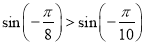B.C.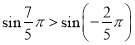D.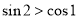数学单选题简单题查看答案及解析

• 已知函数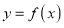在区间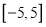上是增函数，那么下列不等式中成立的是（　　 ）

A.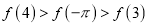B.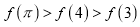C.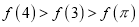D.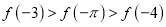数学单选题简单题查看答案及解析

• 下列等式中成立的是（　　 ）

A.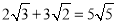B.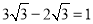C.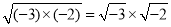D.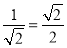八年级数学选择题简单题查看答案及解析

• 已知点C是线段AB的黄金分割点，且AC＞BC，则下列等式中成立的是（     ）

A、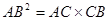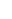B、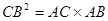C、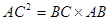D、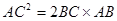八年级数学选择题简单题查看答案及解析

• 如图，一个凸六边形的六个内角都是120°，六条边的长分别为a，b，c，d，e，f，则下列等式中成立的是（　 ）A．a+b+c=d+e+f B．a+c+e=b+d+f C．a+b=d+e D．a+c=b+d

八年级数学选择题困难题查看答案及解析

• 如图，一个凸六边形的六个内角都是120°，六条边的长分别为a，b，c，d，e，f，则下列等式中成立的是（　 ）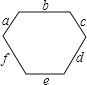A．a+b+c=d+e+f B．a+c+e=b+d+f C．a+b=d+e D．a+c=b+d

八年级数学单选题困难题查看答案及解析

• 若角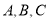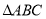的三个内角，则下列等式一定成立的是（　　 ）

A.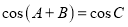B.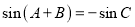C.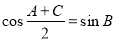D.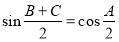高一数学单选题简单题查看答案及解析

• 对可导函数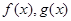,当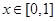时恒有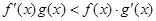.若已知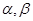是一个锐角三角形的两个内角,且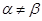,记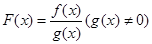.则下列等式正确的是(   )

A.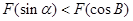B.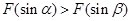C.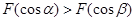D.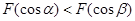高三数学选择题中等难度题查看答案及解析